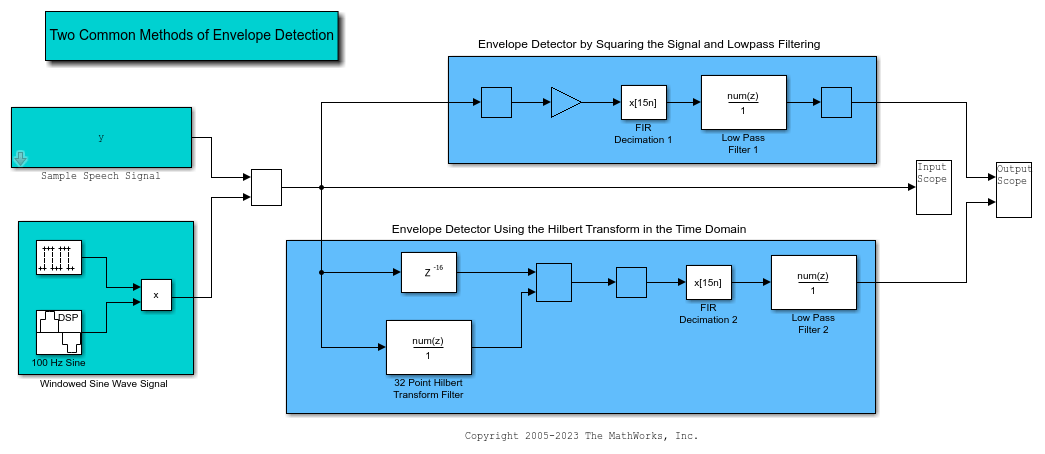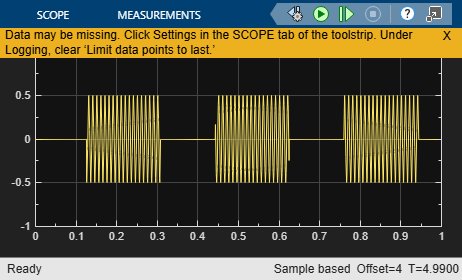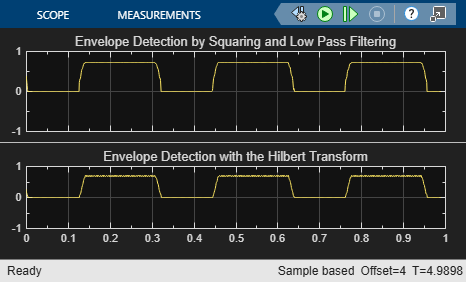This example shows how to implement two common methods of envelope detection in Simulink . One method uses squaring and lowpass filtering. The other uses the Hilbert transform. For a similar example in MATLAB®, see Envelope Detection in MATLAB.

### Method 1: Squaring and Lowpass Filtering

Method 1 works by squaring the input signal and sending it through a lowpass filter. In this Simulink example, a simple minimum-phase lowpass filter is used to remove the high frequency energy. In order to maintain the correct scaling, two more operations are included. The first is to place a gain of 2 on the signal. Since we are only keeping the lower half of the signal energy, this gain boosts the final energy to match its original energy. Finally, the square root of the signal is taken to reverse the scaling distortion from the input signal squaring operation. This method is useful because it is very easy to implement and can be done with a low-order filter, minimizing the lag of the output.

### Method 2: The Hilbert Transform

Method 2 works by creating the analytic signal of the input using a Hilbert transformer. In this Simulink example, the Hilbert transform of the signal is found using a 32-point Parks-McClellan FIR filter. The Hilbert transform of the signal is then multiplied by i (the imaginary unit) and added to the original signal. The original signal is time-delayed before being added to the Hilbert transform to match the delay caused by the Hilbert transform, which is one-half the length of the Hilbert filter. The envelope of the signal can be found by taking the absolute value of the analytic signal. In order to eliminate ringing, and smooth the envelope, apply a lowpass filter to the result. Note that the Analytic Signal block found in the DSP System Toolbox™ could also be used to implement this envelope detection design.

### Envelope Detector Model

The all-platform floating-point version of the Simulink model is shown below. When you run the model, you will see the original signal and the results of both envelope detectors.### Envelope Detector Results

This example shows the results of the two different envelope detectors for two different types of input signals. The input choices are a sample speech signal or a 100 Hz sine wave that turns on and off. The model has a switchable input and two outputs which are routed to scopes for easy viewing. If a signal is not visible, double-click on the Scope block to open it. The input scope plot shows the original signal. The signal lasts a total of 5 seconds, with 1 second of data being shown at a time.

The first output scope plot shows the output of the first envelope detector. This is the result of squaring the original signal and sending it through a low-pass filter. You can clearly see that the envelope was successfully extracted from the speech signal.

The second output scope plot shows the output of the second envelope detector, which employs a Hilbert transform. Though the output of this envelope detector looks very similar to the first method, you can see differences between them.